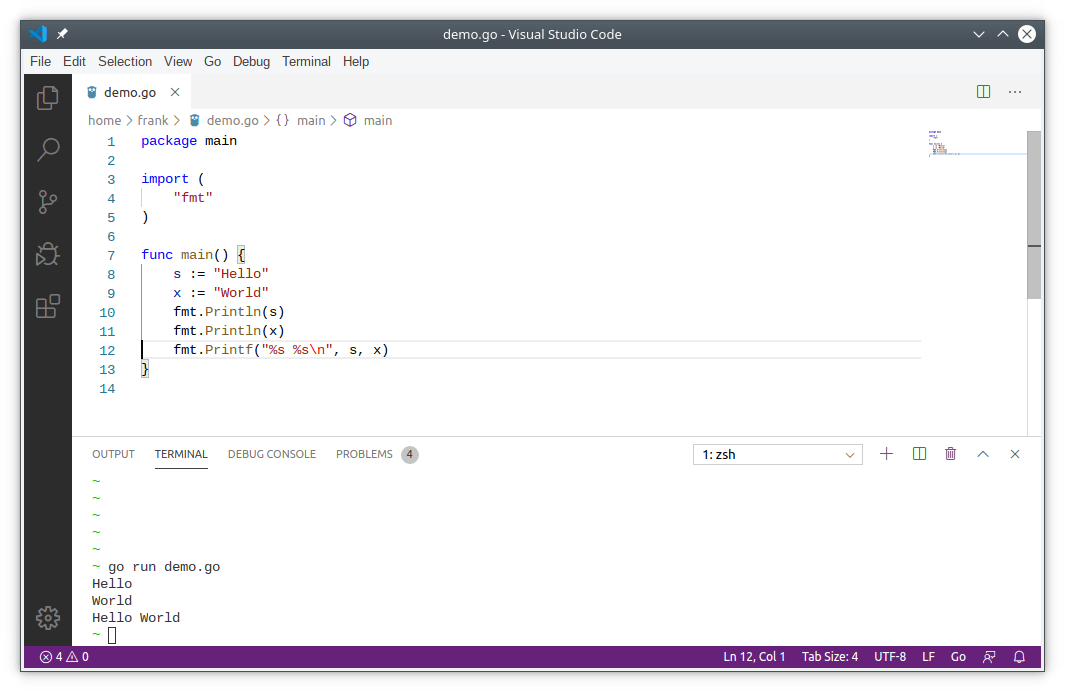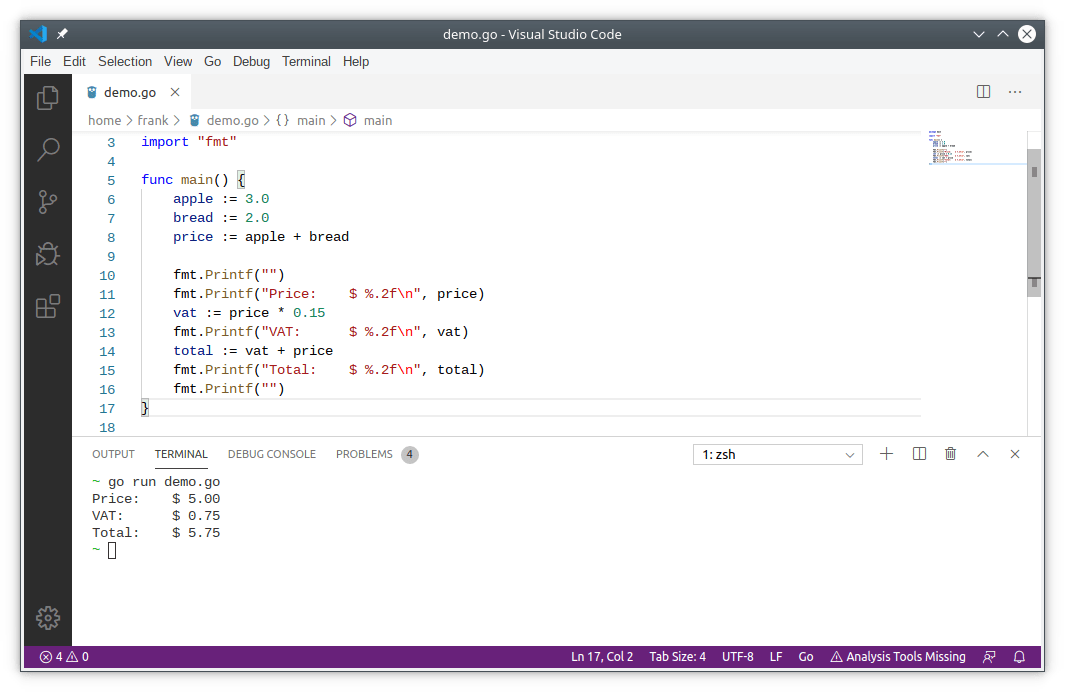Variables often hold text or numeric data. In golang there are several types of variables,
including strings and numeric variables.

Variables can be reused in your code. Arithmetic operations can be used on numeric variables.
String variables can also be changed (sub-strings, concatenation).

## Variables in golang

### Variable declaration

Variables declaration in the Go language: variable names consist of letters, numbers, underscores, where the first letter cannot be numeric.

This type of factoring keyword is generally written to declare global variables and is generally defined outside of func.

When a variable is declared by a var, the system automatically gives it a zero value of that type:

and these variables are initialized in Go.

Note If `//` is used, this means it’s a comment and ignored by Go.

Multiple variables can be assigned on the same line, also known as parallel assignments. For example:

The values on the right of the colon before the equal sign are assigned to the left variable in the same order, so the value of `a` is 5, the value of `b` is 7, and the value of `c` is “abc”.

## go print variables

To output variables you can use the functions `Println` or `Printf`.

This outputs:

## Strings

A string is a text variable. This can be a single character, a word, a phrase, a paragraph or even a book. A string is defined in double quotes `"`, like the strings `s` and `x` below.

To output a single string, you can just pass it into the string function `fmt.Println(x)`. But to output multiple strings you need to use the special symbol %s`.## Numeric variables

Lets start with numeric variables. There are two main types of numeric variables in programming:

• integers (`int`)
• floating points (`float`).

### Integer

An integer is a natural number, like `1,2,3,4`. If you define a variable to be an integer, it cannot be a real number (`1.5, 1.33333`).

To output an integer, you use the symbols `%d`.

Integers use the decimal system (base 10), but you can output integers in other ways like binary (base 2), octal (base 8) or hexadecimal (base 16):

Note: If you don’t know about base 2, base 8 or base 16 don’t worry. You can skip that for now > and just use `%d` for integers.

### Float

A floating point number is not a natural number. You can have values like Pi, `1.3333`, `1.23456` and so on.

Lets do an example. We create a program that calculates the VAT for a given price.

Define a series of products, sum the price ex. VAT, then calculate the VAT and add it to the price. Copy the code below and save the file as `example.go`

To output a `float` (any variable with a dot in the value `.`), you use the symbol `%f`.

Note: All arithmetic operations can be used on variables: division (/), substraction (-), addition (+) and multiplication (*)

In this case it outputs floating point numbers or `float`. This is a number which has numbers behind the dot (behind the comma in europe).

Note: In the above output there are many numbers after the dot. You can limit this by
adding a number. For 2 digits `%.2f`, for 3 digits `%.3f` etc.## Exchange variables

If you want to exchange the values of two variables, you can simply use:

(in Go language, eliminating the need to use the exchange function)

If you run the code, you will see that variable `a` and `b` have changed value.

### blank identifier

The blank identifier is used to abandon the value, such as the value of 5 is abandoned in ` , b = 5, 7.

_ is actually a write-only variable and you cannot get its value. This is done because you must use all of the declared variables in the Go language, but sometimes you do not need to use all the return values you get from a function.

Because the Go language has a mandatory requirement, you must use the declared variable within the function, but the global variable that is not used is no problem.In order to avoid unused variables, the code will fail to compile, and we can change the unused variable to _.

## nil value

The nil designator is used to represent a “zero value” of interface, function, maps, slices, and channels.If you do not specify the type of variable, the compiler will not be able to compile your code because it cannot guess a specific type.

adding an element in a slice of nil is no problem, but doing the same thing to one map will generate a runtime panic:

string is not nil

This is a place that requires attention for developers who often use nil assignment string variables.

`" "` is the zero value of the string and the writing below is the same as the above:

## Video

Video tutorial below:

## Exercises

1. Calculate the year given the date of birth and age
2. Create a program that calculates the average weight of 5 people.